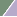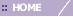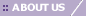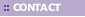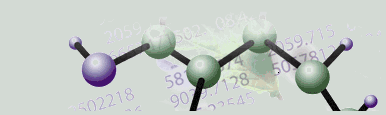# K-Means Clustering Overview

Overview

K-Means clustering generates a specific number of disjoint, flat (non-hierarchical) clusters. It is well suited to generating globular clusters. The K-Means method is numerical, unsupervised, non-deterministic and iterative.

K-Means Algorithm Properties

• There are always K clusters.

• There is always at least one item in each cluster.

• The clusters are non-hierarchical and they do not overlap.

• Every member of a cluster is closer to its cluster than any other cluster because closeness does not always involve the 'center' of clusters.

The K-Means Algorithm Process

• The dataset is partitioned into K clusters and the data points are randomly assigned to the clusters resulting in clusters that have roughly the same number of data points.

• For each data point:

• Calculate the distance from the data point to each cluster.

• If the data point is closest to its own cluster, leave it where it is. If the data point is not closest to its own cluster, move it into the closest cluster.

• Repeat the above step until a complete pass through all the data points results in no data point moving from one cluster to another. At this point the clusters are stable and the clustering process ends.

• The choice of initial partition can greatly affect the final clusters that result, in terms of inter-cluster and intracluster distances and cohesion.

The version of the K-Means algorithm used in GeneLinker™ differs from the conventional K-Means algorithm in that GeneLinker™ does not compute the centroid of the clusters to measure the distance from a data point to a cluster. Instead, the algorithm uses a specified linkage distance metric. The use of the Average Linkage distance metric most closely corresponds to conventional K-Means, but can produce different results in many cases.

• With a large number of variables, K-Means may be computationally faster than hierarchical clustering (if K is small).

• K-Means may produce tighter clusters than hierarchical clustering, especially if the clusters are globular.

• Difficulty in comparing quality of the clusters produced (e.g. for different initial partitions or values of K affect outcome).

• Fixed number of clusters can make it difficult to predict what K should be.

• Does not work well with non-globular clusters.

• Different initial partitions can result in different final clusters. It is helpful to rerun the program using the same as well as different K values, to compare the results achieved.

Note the Warning in Pearson Correlation and Pearson Squared Distance Metric on use of K-Means clustering.

Related Topics:

Distance Metrics Overview